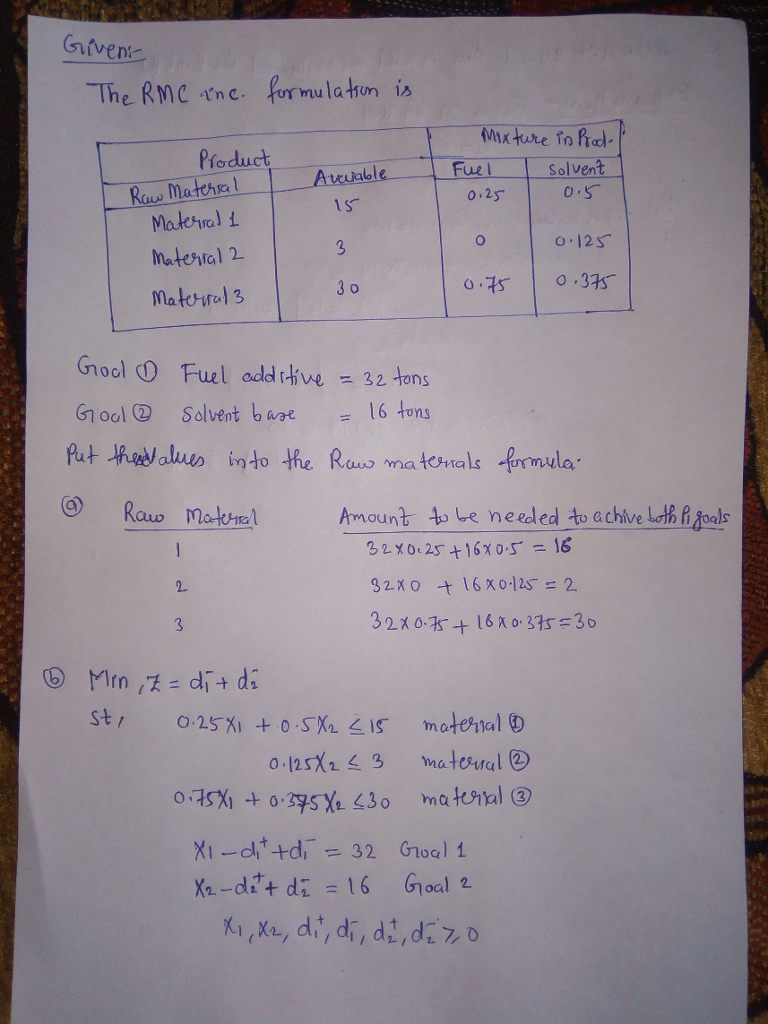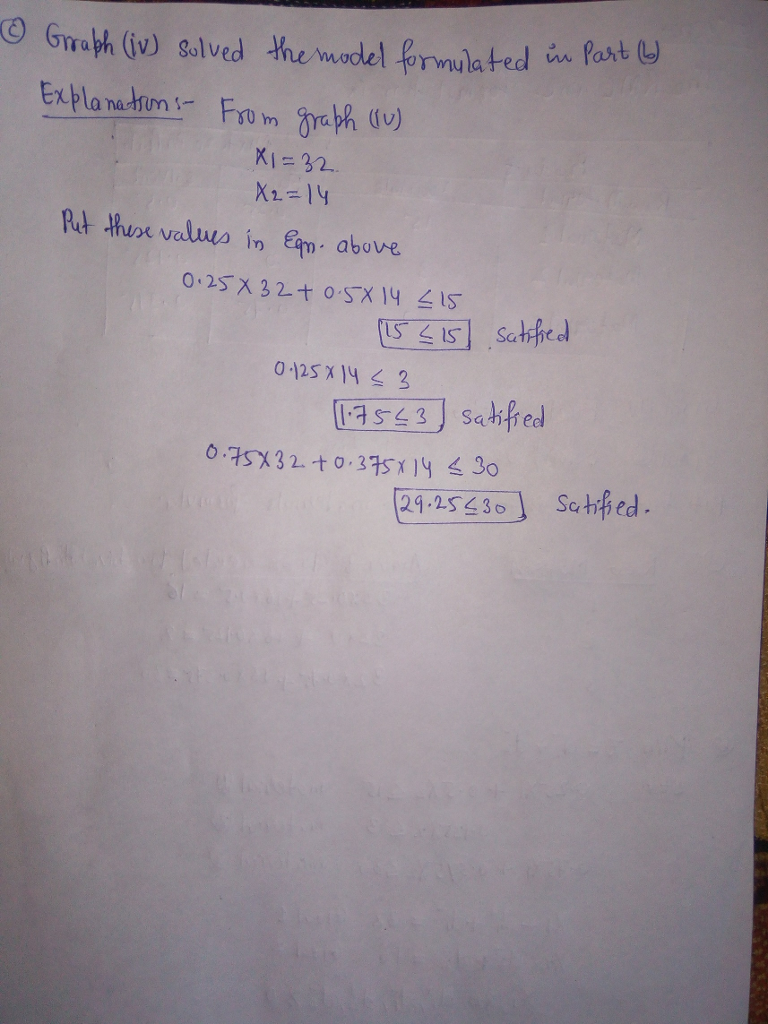# Solved: Problem 14-01 (Algorithmic) The RMC Corporation blends three raw materials to produce

Problem 14-01 (Algorithmic) The RMC Corporation blends three raw materials to produce two products: a fuel additive and a solvent base. Each ton of fuel additive is a mixture of 1/4 ton of material 1 and 3/4 ton of material 3. A ton of solvent base is a mixture of 1/2 ton of material 1, 1/8 ton of material 2, and 3/8 ton of material 3. RMC’s production is constrained by a limited availability of the three raw materials. For the current production period, RMC has the following quantities of each raw material: material 1, 15 tons; material 2, 3 tons; material 3, 30 tons. Management wants to achieve the following P1 priority level goals Goal 1: Produce at least 32 tons of fuel additive Goal 2: Produce at least 16 tons of solvent base Assume there are no other goals a. Is it possible for management to achieve both P1 level goals given the constraints on the amounts of each material available? Amount needed to Raw Material Achieve Both P1 Goals 16 2 30 No, it is impossible b. Treating the amounts of each material available as constraints, formulate a goal programming model to determine the optimal product mix. Assume that both P1 priority level goals are equally important to management. If you don’t need the variable in the model, enter “O”. If you need a negative number, enter minus sign with it. If necessary, enter the numbers as a common fraction Let x1- the number of tons of fuel additive produced X2 = the number of tons of solvent base produced + = the amount by which the number of tons of fuel additive produced exceeds the target value of 32 tons d1-= the amount by which the number of tons of fuel additive produced is less than the target of 32 tons d2+ = the amount by which the number of tons of solvent base produced exceeds the target value of 16 tons d2 = the amount by which the number of tons of solvent base is less than the target value of 16 tons